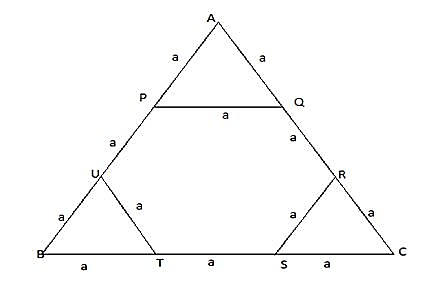# Corners are cut off from an equilateral triangle - Quantitative Aptitude – Geometry | Mensuration |

## CAT 2019 – Slot 1 - CAT Mensuration - Question 5 - Corners are cut off from an equilateral triangle

Q. 5: Corners are cut off from an equilateral triangle T to produce a regular hexagon H. Then, the ratio of the area of H to the area of T is

1. 3 : 4
2. 2 : 3
3. 5 : 6
4. 4 : 5

In given case , figure can be drawn as belowLet side of equilateral triangle ABC = 3a

So side of hexagon = a

Area of triangle = (root3 /4 )*(3a)^2 = 9a^2 *(root3/4)

Area of hexagon with side a = 6*(root3 /4 )*(a)^2

Ratio of areas of hexagon to that of triangle = { 6*(root3 /4 )*(a)^2 } / { 9a^2 *(root3/4)} = 6/9 = 2/3

Thus required ratio = 2 : 3

### Past Year Question Paper & SolutionsCounselling Session
By IIM Mentor

#### Free Material Area